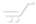# Operators precedence.

Operators precedence.
Operators precedence is the order in which NumericBase performs operations in formulas.

If you combine several operators in a single formula, NumericBase performs the operations in the order shown in the following table.

In this table, an earlier location means higher precedence. For example, the member access operators has the highest precedence so they are evaluated first.

 Operators Meaning . [ ] @ member access operators such as in Table.a and a - Negation (as in -1). * / Multiplication and division + - Addition and subtraction. & Connects two strings of text (concatenation). = < > <= >= <> Comparison. not Logical operator. and Logical operator. or Logical operator. if then else The if operator. alt The "alt" operator
Table: Operators precedence.

operators with the same precedence
If a formula contains operators with the same precedence - for example, if a formula contains both a multiplication and division operator - NumericBase evaluates the operators from left to right.

Using parenthesis
To change the order of evaluation, enclose the part of the formula to be calculated first in parentheses.